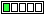All about flooble | fun stuff | Get a free chatterbox | Free JavaScript | Avatarsperplexus dot infoA missing symbol (Posted on 2014-01-08)3*5=6

The above statement is not true, but you could make it true just by inserting a certain mathematical symbol into this equation in three different places.

Same symbol!

What is this symbol?

 See The Solution Submitted by Ady TZIDON No RatingComments: ( Back to comment list | You must be logged in to post comments.)Some solutions| Comment 1 of 2
You could add the symbol 0 as an exponent three times.  Pretend the degree sign is a zero:  3�*5�=6�

You could also write three 0's to the lower left of the numerals to make them appear as exponents:
0^3*0^5=0^6
Or use 1's
1^3*1^5=1^6

If you allow the differential operator d/dx as a single symbol you get
d3/dx*d5/dx=d6/dx
which is true because 0*0=0
If you use the prime notation for a derivative this is the same as
3'*5'=6'

Is this is a 'word problem'?
I can't think of solutions for
THREE TIMES FIVE EQUALS SIX

 Posted by Jer on 2014-01-08 11:32:52Please log in:

 Search: Search body:
Forums (0)